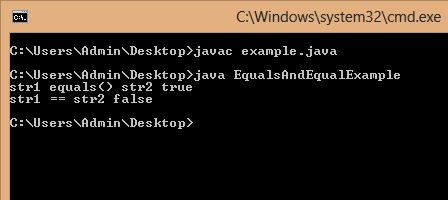# Difference Between equals() and == in Java

The equals() method and == operator in Java perform two different operations. The equals() method is used to compare two string values. On the other hand == operator is used to compare two primitive datatype variables or to compare references of two objects. Below is a sample program that shows difference between equals() and == in Java.

OutputIn first case we are comparing the values or content of String objects str1 and str2 using equals() method. The result is true because there values are equal.

In the second case we are comparing the references of String objects str1 and str2 using == operator. The result is false because there references are not equal as both the objects are different.

### 3 thoughts on “Difference Between equals() and == in Java”

1.This is not correct in Java 8. Equal and “==” is same when compare String objects in Java 8.
This issue just happen in past

1.I dont think so, can u give any example that give two different results in Java 8 and previous versions.

2.How to validate 12 digit alphanumeric number in Java.
Ex. US12345678A1
In this string first 2 characters must always be alphabet.
And next 9 characters must be alphanumeric means must contains both number and alphabet.
Last one must always be numeric.